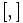# LCS-Baer correspondence

## Definition

This is a generalization of the Baer correspondence between some groups of nilpotency class at most two and some Lie rings of nilpotency class at most two.

LCS-Baer Lie rings$\leftrightarrow$ LCS-Baer Lie groups

The Lie rings in question are LCS-Baer Lie rings. A LCS-Baer Lie ring is a Lie ring of class at most two with the property that its derived subring is 2-powered, i.e., every element has a unique half. In particular, this includes both abelian Lie rings (where the derived subring is the zero subring) and Baer Lie rings (groups of class at most two where the whole Lie ring is uniquely 2-divisible).

The groups in question are LCS-Baer Lie groups. A LCS-Baer Lie group is a group of class at most two with the property that its derived subgroup is 2-powered, i.e., every element of the derived subgroup has a unique square root in the derived subgroup. In particular, this includes both abelian groups (where the derived subgroup is trivial) and Baer Lie groups (groups of class at most two where the whole group is uniquely 2-divisible)

### From group to Lie ring

Suppose$G$ is a LCS-Baer Lie group. In particular, this means that the derived subgroup of$G$ is contained in the center of$G$ and that every element in the derived subgroup has a unique square root in the derived subgroup. Denote by$\sqrt{}$ the function from$[G,G]$ to itself that sends an element in the derived subgroup to its unique square root in the derived subgroup.

Lie ring operation that we need to define Definition in terms of the group operations Further comments
Addition, i.e., define$x + y$ for$x,y \in G$$x + y := \frac{xy}{\sqrt{[x,y]}}$ By definition,$\sqrt{[x,y]} \in [G,G]$ as well, and is hence also in the center. Thus, to divide by it, we do not need to specify whether we are dividing on the left or on the right.
Identity element for addition, denoted$0$. Same as identity element for group multiplication, denoted$e$ or$1$. This automatically follows from the way addition is defined.
Additive inverse, i.e., define$-x$ for$x \in G$. Same as$x^{-1}$, i.e., the multiplicative inverse in the group. This automatically follows from the way addition is defined.
Lie bracket, i.e., the$[ , ]$ map in the Lie ring. Same as the group commutator$[x,y] = xyx^{-1}y^{-1}$.

### From Lie ring to group

Suppose$L$ is a LCS-Baer Lie ring, with addition denoted$+$ and Lie bracket denoted$[ , ]$. Denote by$\frac{1}{2}$ the operation from$[L,L]$ to itself sending each element to its unique half. We give$L$ the structure of a class two group as follows:

Group operation that we need to define Definition in terms of the Lie ring operations Further comments
Group multiplication$xy := x + y + \frac{1}{2}[x,y]$ By definition,$[x,y] \in [L,L]$ so$\frac{1}{2}[x,y]$ makes sense.
Identity element for multiplication Same as the zero element$0$ of the Lie ring.
Multiplicative inverse$x^{-1}$. Same as the additive inverse$-x$.
Group commutator$[x,y] = xyx^{-1}y^{-1}$ Same as the Lie bracket$[x,y]$.

## Examples

In the case of an abelian group, the corresponding Lie ring is an abelian Lie ring with the same group as additive group, and the mapping is an identity mapping.

In the case of a Baer Lie group, such as an odd-order class two group, the corresponding Lie ring is the corresponding Baer Lie ring and we get a Baer correspondence.

A finite nilpotent group is a LCS-Baer Lie group if its 2-Sylow subgroup is abelian and all other Sylow subgroups have class at most two.